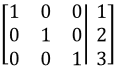# Representing a linear system as a matrix

### Representing a linear system as a matrix

In this lesson, we will learn how to turn a linear system into a matrix. What we do is draw a big bracket, take all the coefficients of each term and write it in, draw a vertical line, write all the numbers after the equal sign, and end it with another big bracket. Terms that do not seem to have a coefficient actually do. For example the term y can be rewritten to 1*y, and so the coefficient of this will be 1. Notice that when you turn it into a matrix, all the variables disappear since the most important part are the numbers.
Basic Concepts: Notation of matrices

#### Lessons

We can represent a linear system as a matrix. For example, the linear system

$1x+2y+3z=4$
$5x+6y+7z=8$
$9x+10y+11z=12$

can be represented as the matrix: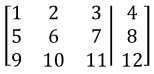where $x,y,z$ are variables and the vertical line represents the equal sign for each linear equation. We see all the $x,y,z$'s disappear, and we take all the coefficients and the numbers after the equal sign.
• Introduction
Representing a linear system as a matrix Overview:

• 1.
Representing a linear system as a matrix
Represent each linear system as a matrix:
a)
$x+6y-3z=3$
$4x+2y-z=10$
$6x+10y+20z=0$

b)
$-3x+7y=10$
$10x+2y=15$

c)
$v+w+x+y+z=0$
$x+y+z=5$
$x+y=3$
$2v+4w=2$
$y=3$

d)
$9x=3$

e)
$2w+6y+2z=3$
$3x+6y=2$
$x+y+z=10$
$w+2x+10y+z=7$

• 2.
Representing a matrix as a linear system
Represent each matrix as a linear system:
a)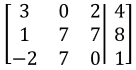b)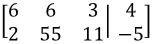c)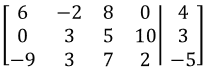d)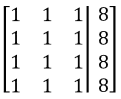e)